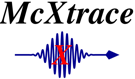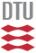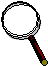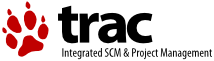McXtrace: Saxs_spheres Component

# The `Saxs_spheres` Component

Sample for Small Angle X-ray Scattering - hard spheres in thin solution, mono disperse.

## Identification

• Author: E. B. Knudsen, P. Willendrup, K. Lefmann, L. Arleth
• Origin: DTU Fysik
• Date: 28.10.2010
• Version: beta

## Description

```Sample for use in a SAXS instrument, models hard, monodisperse spheres in thin solution.
The shape of the sample may be a filled box with dimensions
xwidth, yheight, zdepth, a cylinder with dimensions radius and yheight,
a filled sphere with radius R.

Example: Saxs_spheres(R = 20, Phi = 1e-3, Delta_rho = 0.6, sigma_abs = 50, xwidth=0.01, yheight=0.01, zdepth=0.005)

```

## Input parameters

Parameters in boldface are required; the others are optional.
Name Unit Description Default
sphere_mtrl NULL
R AA Radius of scattering hard spheres 100
Phi 1 Particle volume fraction 1e-3
Delta_rho fm/AA^3 Excess scattering length density 0.6
xwidth m horiz. dimension of sample, as a width 0
yheight m vert . dimension of sample, as a height for cylinder/box 0
zdepth m depth of sample 0
radius m Outer radius of sample in (x,z) plane for cylinder/sphere 0
target_x - 0
target_y m position of target to focus at 0
target_z - 6
target_index 1 Relative index of component to focus at, e.g. next is +1 0
focus_xw m horiz. dimension of a rectangular area 0
focus_yh m vert. dimension of a rectangular area 0
focus_aw deg horiz. angular dimension of a rectangular area 0
focus_ah deg vert. angular dimension of a rectangular area 0
focus_r m Detector (disk-shaped) radius 0
mu_c 5 Column of the datafiel which contains absorption coefficients. 0

## Output parameters

Name Unit Description Default
shape
prms
prmsp